# One-hot encoding

## Introduction

Sometimes when working with datasets, we encounter columns that have categorical variables which cannot be processed efficiently by our model. Let’s consider a dataset of Houses in 4 Areas in Delhi Namely ‘Greater Kailash’, ‘Civil Lines’, ‘Ashok Vihar’, and ‘Pitampura’. These string variables cannot be processed efficiently by the training model. Hence we need to change this column into a more machine-friendly data format. This can be done with an encoding technique called One-Hot Encoding.

## Why one-hot encoding?

Categorical data encoding is an essential and unavoidable step in data preprocessing. There are various techniques for data encoding. One such technique is one-hot encoding. Let us take the gender feature example again. For every entry in the dataset, we have a gender associated with it, either ‘Male’ or ‘Female’ (assuming there are no empty values). These string values need to be converted to numerical values for our model to make use of the feature.

Let’s say we label encode the gender feature where ‘Male’ is assigned 1 and Female is assigned 0 as shown below.Now, We may have converted string variables to corresponding machine-friendly numerals. But as humans we know, there is no specific order in this. That 1 for ‘Male’ is just a numeric representation of the string. However, while training, the machine may assign a higher weight to datapoints with Gender value as ‘1’ compared to those with ’0’. But genders themselves don’t have any hierarchical order whatsoever. Hence Label encoding isn’t the best way to categorically encode features that don’t have any specific order among themselves. It’s best to use One hot encoding in this case.

However, in case of ordered categorical features, Label encoding may make more sense. Suppose we have a dataset of athletes where we have ‘Pace’ as a feature with Categorical variables as ‘Low’ ,’ Medium’, and ‘High’.

In this case we might assign

Low : 0

Medium : 1

High : 2

Since the variables themselves have some order.

## One-Hot Encoding

One hot encoding is a data encoding technique that creates a feature for every unique value in the column. These columns are called dummy variables. This will create both ‘Male’ and ‘Female’ columns for every entry in the dataset. So suppose for an entry we have the gender feature as ‘male’. This will enable the ‘Male’ column with 1 and the ‘Female’ column would still be 0.

Now let us consider a bit more complex example. Suppose we have a dataset of animals where we have a feature called ‘Species’. Let’s say our dataset has only 5 types of animal species namely ‘Dogs’, ‘Cats’, ‘Sheep’, ‘Horse’, and ‘Lion’. After one-hot encoding, our table will look like this -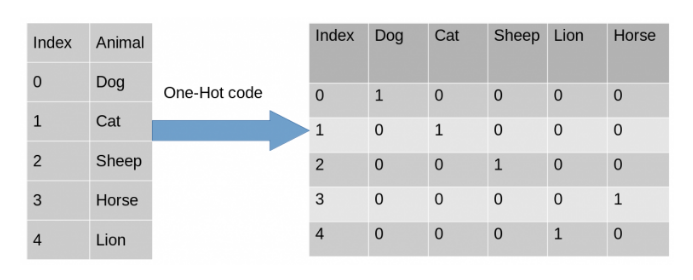We have 5 new dummy variables for every unique species of animal. This helps us efficiently categorize our features.

## Implementation

Let us implement one-hot encoding in python. You can find the dataset here

There are 2 popular methods to implement one-hot encoding on categorical features using pandas as well as sklearn libraries. We will look at both these approaches.

### Using pandas

``````import pandas as pd
import numpy as np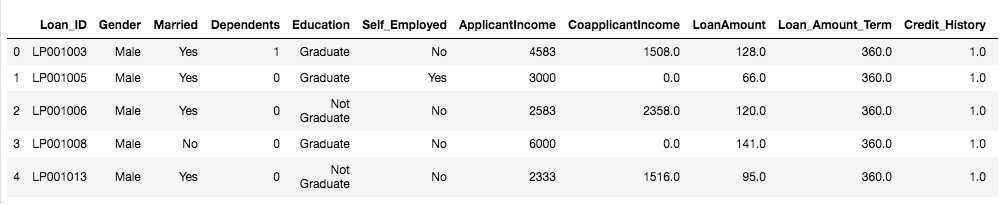``````X = data[['Education','Married']]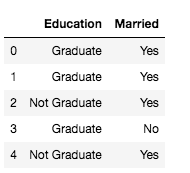``````print(X['Education'].unique())
print(X['Married'].unique())``````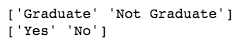``````one_hot_encoded_data = pd.get_dummies(X, columns = ['Education', 'Married'])
print(one_hot_encoded_data)``````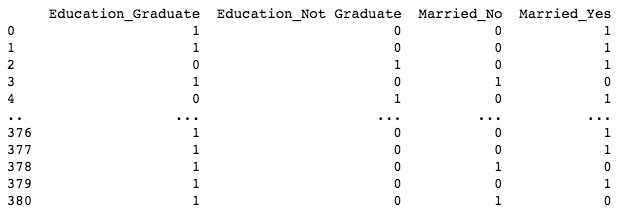### Using Sklearn

Using sklearn one hot encoder, we first label encode the categorical variables

``````from sklearn.preprocessing import LabelEncoder
# creating initial dataframe
Ed_df = pd.DataFrame(X, columns=['Education'])
# creating instance of labelencoder
labelencoder = LabelEncoder()
# Assigning numerical values and storing in another column
Ed_df['Ed_Types_Cat'] = labelencoder.fit_transform(Ed_df['Education'])
Ed_df``````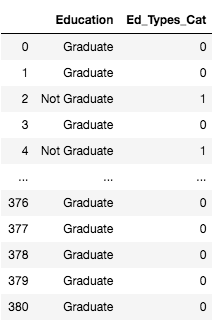Here, since we have only 2 variables in the Education column, we generate 2 label encoded variables 0 and 1.

``````from sklearn.preprocessing import OneHotEncoder
# creating instance of one-hot-encoder
enc = OneHotEncoder(handle_unknown='ignore')
# passing bridge-types-cat column (label encoded values of bridge_types)
enc_df = pd.DataFrame(enc.fit_transform(Ed_df[['Ed_Types_Cat']]).toarray())
# merge with main df bridge_df on key values
Ed_df = Ed_df.join(enc_df)
Ed_df``````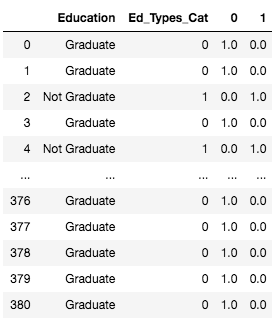We then make dummy variables from the generated labels and then one hot encode the feature.

We can then concatenate the new data frames to the original data frame for the training phase.

1. Why do we need to encode the categorical features of the dataset?
Ans. The string variables in the categorical feature column can’t be processed efficiently by our model. Hence it’s essential to map them to some numeric value.

2. Mention some of the data encoding techniques.
Ans. One-hot encoding, Label encoding, Dummy encoding, Hash encoding.

3. Briefly explain one-hot encoding.
Ans. One-hot encoding creates dummy variables for every unique value in the categorical feature column. The dummy variable values are then mapped to the dataset.

## Key takeaways

Data processing is essential before developing any machine learning model. Encoding the categorical data is a vital step in the data processing. One hot encoding is a very commonly used encoding technique. Therefore it’s essential to have in-depth knowledge about it and where it can be employed. You can check out our expert-curated data science course if you want to prepare for your next data science interview.

Happy Learning!!